# 第五章：图（图的应用）

## 1.最小生成树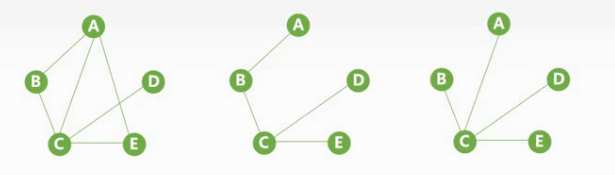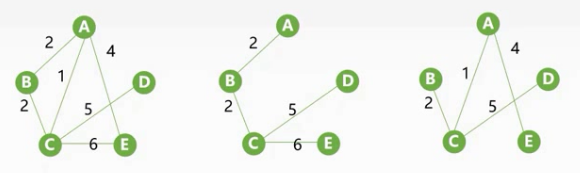### 1.1性质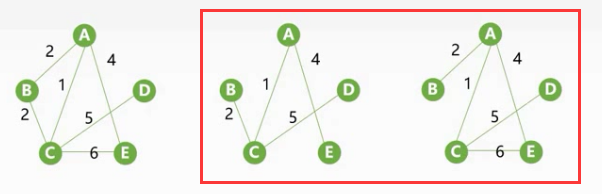• 所有边的权重皆不相同（没有权重相同的边最小生成树必为一，可以自行尝试）
• 图中n个顶点只有n-1条边（这样的无向图【网】它的最小生成树只有一个就是他自己，所以唯一）

1. 最小生成树不一定唯一,即最小生成树的树形不一定唯一。当带权无向连通图G的各边权值不等时或G只有结点数减1条边时,MST唯一

2. 最小生成树的权值是唯一的,且是最小

3. 最小生成树的边数为顶点数减1

### 1.2算法

//伪代码
GENRIC_MST(G){
T=NULL;
while T //未形成一棵生成树
do //找到一条最小代价边(u,v)并且加入T后不会产生回路
T=Tu(u,v);
}


### 1.3Prim

{(u,v)|u∈Vt,v∈V-Vt} 相当于将已经添加到结果集中的结点和未添加进来的结点进行连接，这种方法就避免了生成的生成树出现回路

Vt=VtU{v},Et=EtU{(u,v)} 将新添加的顶点添加到结果的顶点集中，边添加到结果的边集中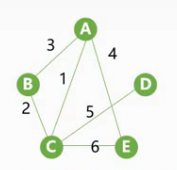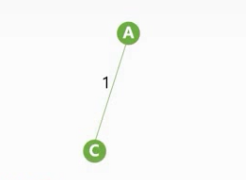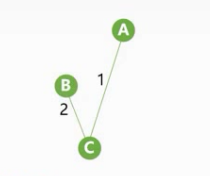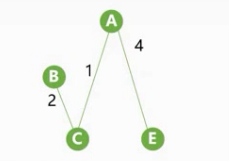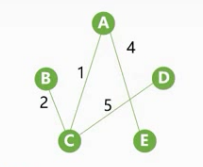void Prim(G,T){
T = 空集;//结果集
U = {w};//将w顶点加入结果的顶点集中
while((V-U)!=空集){
设（u,v）是使u∈(V-U)，且权值最小的边；
T=TuP{(u,v)};
U=Uu{V};
}
}


• min_weight[n] 它的数组大小是顶点的数量大小，他代表我们每次循环时，我们知道我们的边一个顶点要从结果集中选择另一个顶点要从剩下的顶点中选择，它存放了到每一个顶点：我们已经生成的顶点到我们剩下的顶点的权值最小的那条边的，数组下标是被选择的顶点的下标，例如min_weight代表我们已经挑选的顶点到剩下的顶点之前权重最小的那一条边，下标2则代表结果顶点集中的顶点到剩下的顶点中下标2的顶点。
• adjvex[n] 存放该顶点由那一个顶点引入结果集中的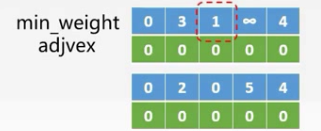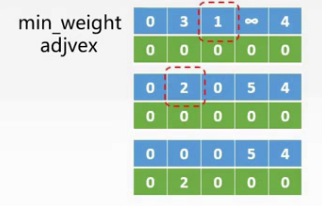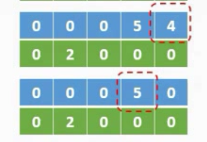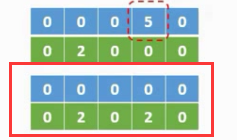void MST_Prim(Graph G){ //传入图G
int min_weight[G.vexnum];//数组大小为顶点的数量
for(int i=0; i<G.vexnum;i++){
//可以看到初始顶点是下标为0的顶点，且可以看出我们的顶点这里采用的是邻接矩阵存储的
min_weight[i]=G.Edge[i];//存放权值
}
int min_arc; //存放当前挑选的最小边的权重
int Min_vex; //挑选这样的一条边的另一个端点的数组下标
for(int i=1;i<G.vexnum;i++){//循环剩余顶点注意这里i初始值为1，因为我们已经挑选一个了
min_arc=MAX;//将最小边的权重置为max，这里是为了求最小值的时候可以进行比较
for(int j=1;j<G.vexnum;j++){//挑选满足条件的边
//min_weight[j]!=0不等于0代表这个顶点还没有被添加到结果集
//min_weight[j]<min_arc 判断权值是否是最小的
if(min_weight[j]!=0&&min_weight[j]<min_arc){
min_arc=min_weight[j];//如果小于之前的权值，修改最小的权值
min_vex=j;//同时保存最小权值结点的下标
}
}
min_weight[min_vex]=0;//表示已经该顶点已经被选择出来了
for(int j=0;j<G.vexnum;j++){//循环修改对应数组下标下辅助变量的值
//min_weight[j]!=0不等于0代表这个顶点还没有被添加到结果集
//G.Edge[min_arc][j]<min_weight[j] 因为结果集中加入了新顶点，所以最小的权值可能会发生改变
//所以我们需要修改辅助数组的权值
if(min_weight[j]!=0&&G.Edge[min_arc][j]<min_weight[j]){
min_weight[j]=G.Edge[min_arc][j];//如果加入的这条边的权值比之前的权值小则替换之前的
}
}
}
}


### 1.4Kruskal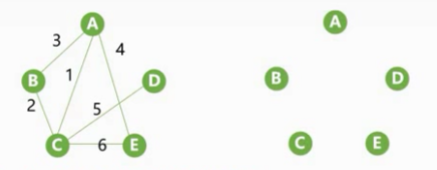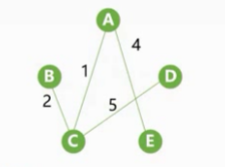Kruskal主要使用了堆排序对边的权重进行排序，然后使用并查集(数组)这个数据挑选边。代码实现

typedef struct Edge{
int a,b;//改变两个端点的下标
int weight;//权重
};
void MST_Kruskal(Graph G,Edge *edges,int *parent){//图G、属于该图的边的集合、并查集辅助变量
heap_sort(edges);//堆排序
Initial(parent);//初始化并查集
for(int i=0;i<G.arcnum;i++){//循环次数为边的数目
int a_root=Find(parent,edges[i].a);//求第一个端点所在并查集的根结点的下标
int b_root=Find(parent,edges[i].b);//求第而个端点所在并查集的根结点的下标
if(a_root!=b_root){//如果不相同
Union(parent,a_root,b_root);//并入结果集
}
}
}


## 2.最短路径

### 2.1Dijkstra(迪杰斯特拉)

Dijkstra(迪杰斯特拉)：计算带权图单源最短路径。我们需要以下几个辅助数组：

• s[] 标记已经计算完成的顶点：数组中的值全部初始化为0(未计算完成)，源点下标的值初始化为1(计算完成)
• dist[] 记录从源点v0到其他各顶点当前的最短路径长度：数组中的值初始化为源点到各个顶点边的权值，即dist[i]=arcs[i] (我们这里源点是0，所以从第0行取值初始化，这样看源点的变化)
• path[] 记录从最短路径中顶点的前驱顶点，即path[i] 为v到vi最短路径上vi的前驱顶点（最后我们不断的求前驱系结点组成的就是一条最短路径）：数组中值的初始化，若源点v0到该顶点vi有一条有向边(无向边)，则令path[i]=0，否则path[i]=-1

• 初始化数组，并集合S初始化为{0}(令源点为v0)
• 从顶点集合V-S中选出Vj，满足dist[j]=Min{dist[i],vi∈V-S}，Vj就是当前求得的最短路径的终点，并令S=S∪
• 修改此时V0出发到集合V-S上任一顶点Vk的最短路径的长度：若dist[j]+arcs[j][k]<dist[k]则令dist[k]=dist[j]+arcs[j][k];path[k]=j;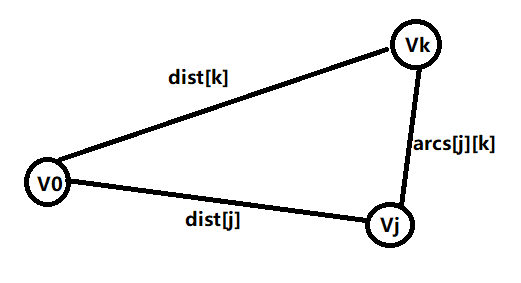• 重复 2、3操作n-1次，直到S中包含全部顶点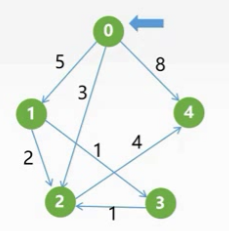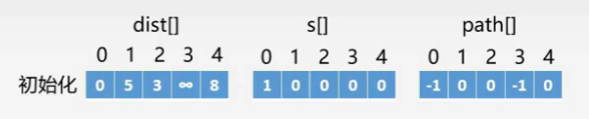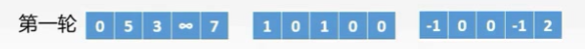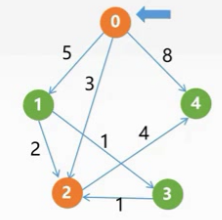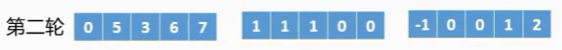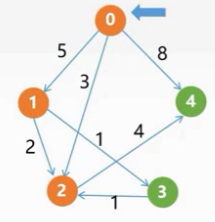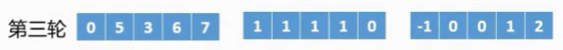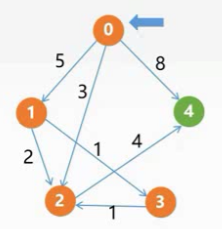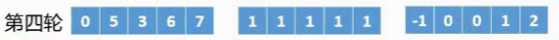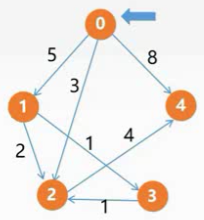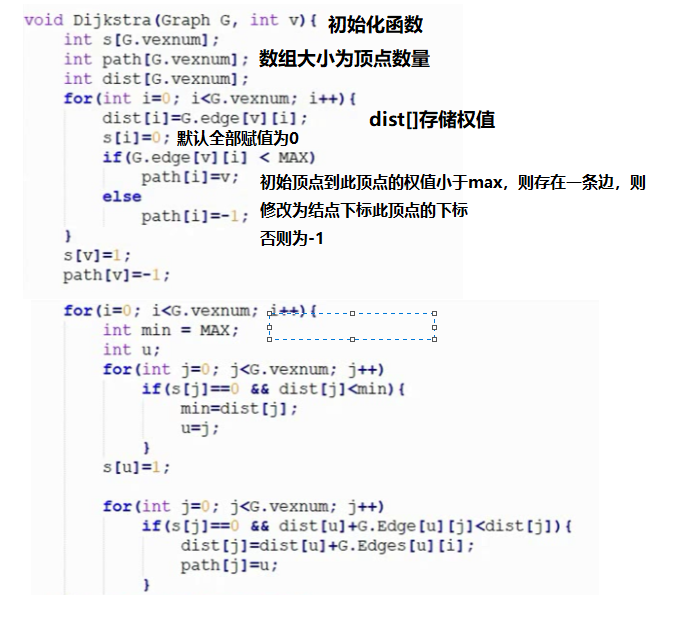### 3.2Floyd(弗洛伊德算法)

Floyd(弗洛伊德算法)：计算各个顶点之间的最短路径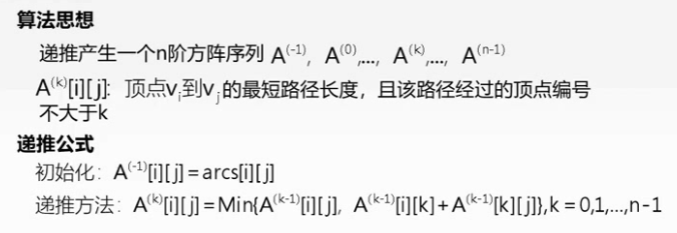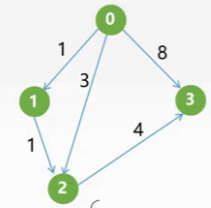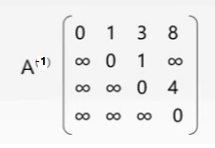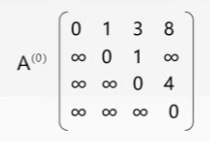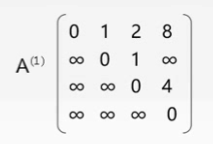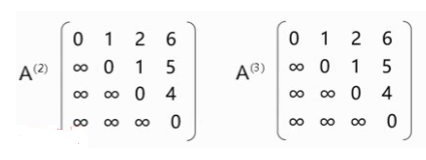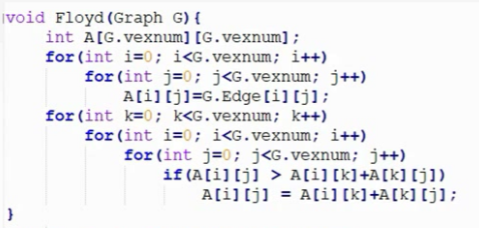## 3.拓扑排序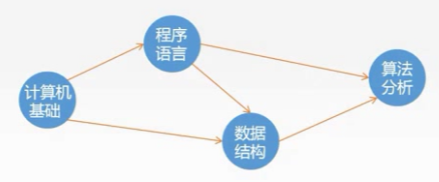有向无环图：不存在环的有向图,简称DAG图。

AOV网：若用一个.AG图表示一个工程，其顶点表示活动，用有向边<vi,vj>表示活动 vi 先于活动 vj 进行的传递关系，则将这种DAG称为顶点表示活动网络，记为AOV网。(虽然它叫网，但是它的边是没有权值的)

拓扑排序：对DAG所有顶点的一种排序，使若存在一条从顶点A到顶点B的路径，在排序中B排在A的后面

算法思想

• 1)从DAG图中选择一个没有前驱的顶点并输出

• 2)从图中删除该顶点和所有以它为起点的有向边

• 3)重复1)、2)直到当前的DAG图为空或当前图中不存在无前驱的顶点为止。后一种情况说明图中有环(则不是有向无环图)。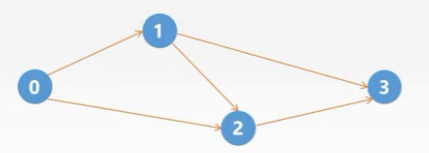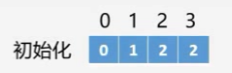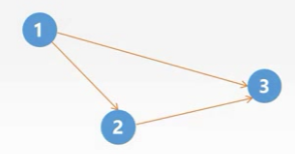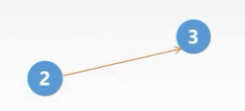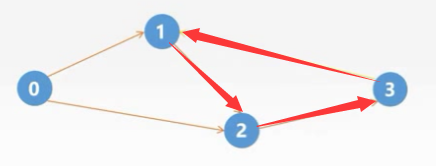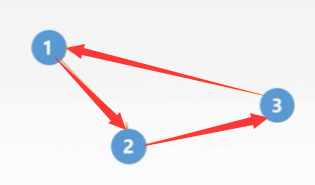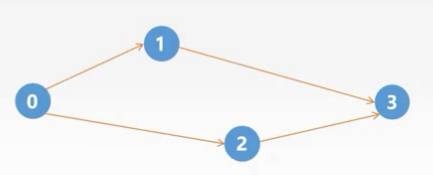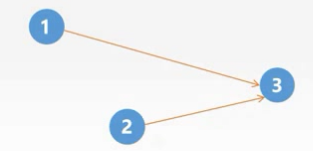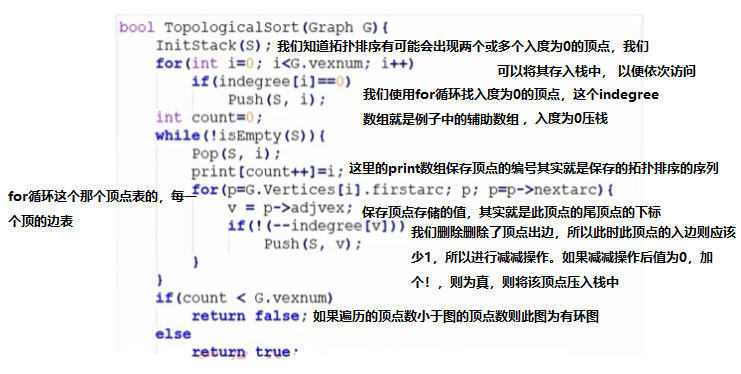• 若邻接矩阵为三角矩阵，则存在拓扑排序；反之不一定成立

## 4.关键路径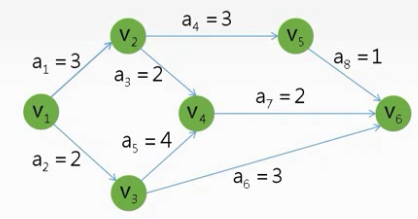AOE网：在有向带权图中，以顶点表示事件，以有向边表示活动，以边上权值表示完成该活动的开销(如完成活动所需要的时间)，则称这种有向图为用边表示活动的网络，简称AOE网

AOE网有以下特点

• 它始终只有一个顶点是完全没有入边的，我们称为源点，如上图的V1
• 它始终只有一个顶点是完全没有出边的，我们称为汇点，如上图的V6

关键路径：从原点到汇点最大路径长度的路径（权重和最大的路径）称为关键路径，关键路径上的活动为 关键活动

### 4.1关键路径的计算1、事件Vk的最早发生时间Ve(k)

Ve(源点)=0; Ve(k)=Max{Ve(j)+Weight(Vj,Vk)}

Weight(Vj,Vk)：顶点Vj到当前顶入边的权值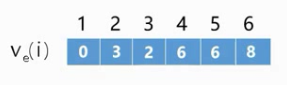2、事件Vk的最迟发生时间Vl(k)

Vl(汇点)=Ve(汇点);//令汇点的最迟发生时间等于汇点的最早发生时间，如果我们汇点的最迟发生时间大于汇点的最早发生时间那么我们就等于推迟了工程的结束时间，所以只能相等

Vl(j)=Min{Vl(k)-Weight(Vj,Vk)} 我们计算最早发生时间使用了入边，这里我们使用出边向源点进行逆推。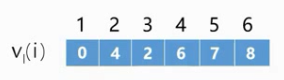3、活动ai的最早开始时间e(i)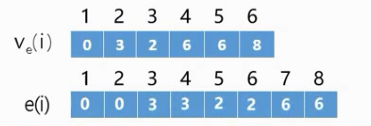4、活动ai的最迟开始时间l(j)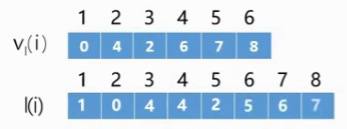5、活动ai的差额d(i)=l(i)-e(i)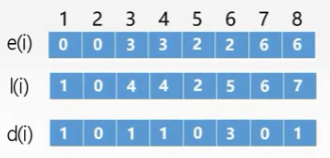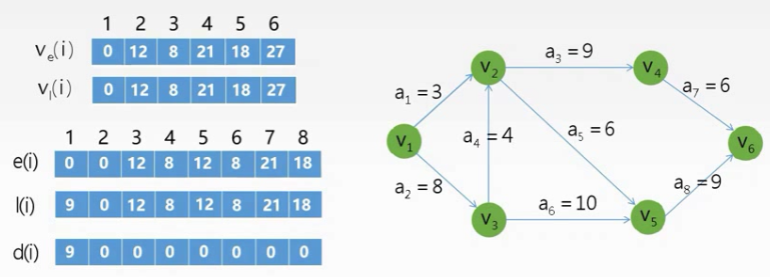## 7.理木客